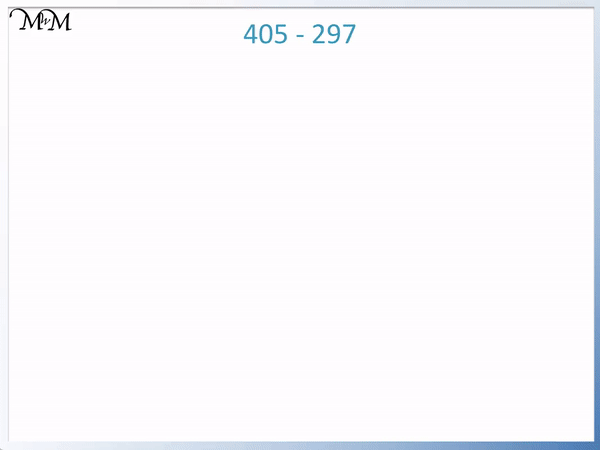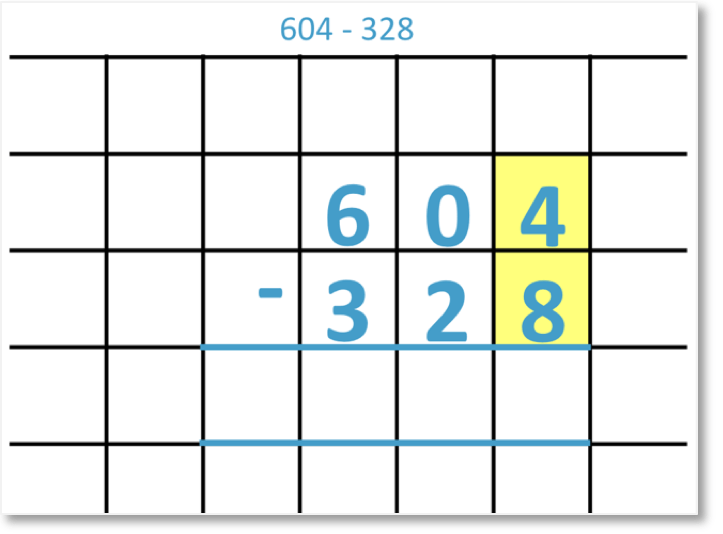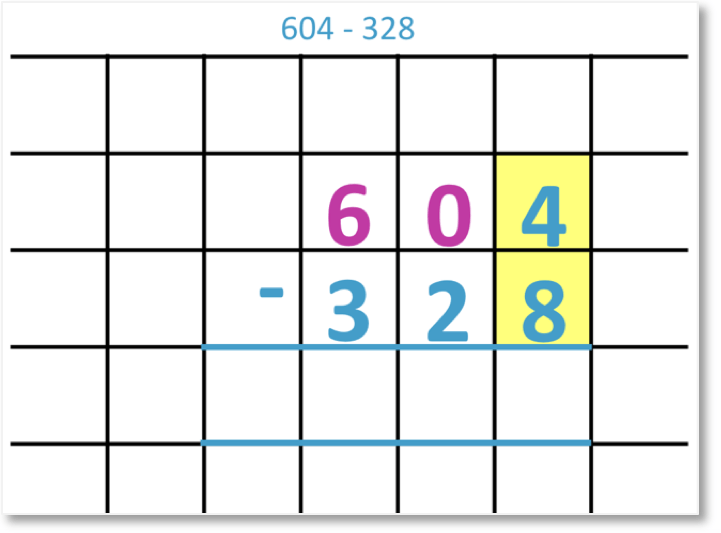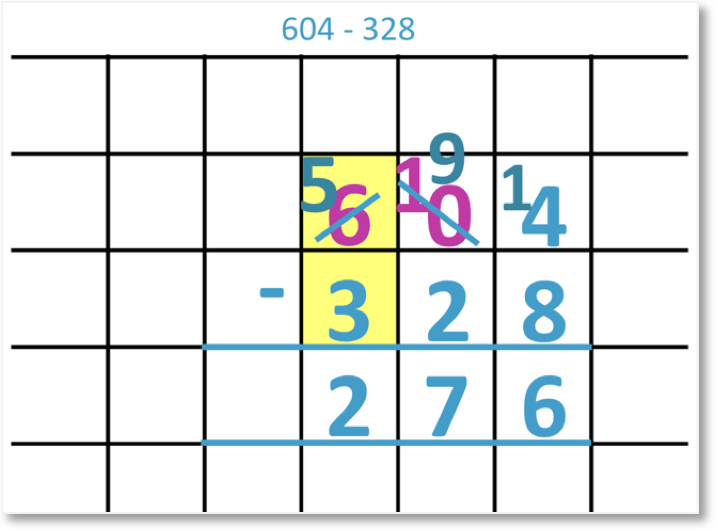# Column Subtraction Borrowing from Zero

Column Subtraction Borrowing from Zero• In the column subtraction of 405 – 297 we need to borrow from the tens column since 7 is greater than 5.
• However we have a zero in the tens column.
• We first borrow a hundred from the ‘4’ in the hundreds column and regroup it to the tens column to make the ‘0’ into a ’10’.
• We can now borrow from this ’10’ in the tens column and carry a one into the units column to make ’15’.
• We can now subtract all columns because the numbers we are subtracting from are now all larger.

#### Alternate MethodWe can treat the ‘400’ as ’40’ tens’ and borrow a ten immediately to leave ’39’ tens in total.Supporting Lessons# Column Subtraction Borrowing From Zero

In this lesson we will be learning how to use the column subtraction method when we need to borrow from zero. These examples will be of column subtraction with zero in the middle of a three digit number.

We will look at how to calculate 604 – 328. Here we are subtracting from a three-digit number with zero in the middle.We begin by lining up the digits in their place value columns. We want to subtract the digits in the units column. However, 8 is greater than 4, so we can’t.We would normally borrow (regroup) from the tens column. However, we have a zero written here, which means that there aren’t any tens.Therefore, before we can borrow some units from the tens, we need to borrow some tens from the hundreds column. We need to borrow from the 6 hundreds.We move 1 hundred next to the zero to make 10 tens. We are left with 5 hundreds. This process is also known as regrouping.Now that we have 10 tens, we can borrow some units from this column. We move 1 ten next to the 4 to make 14 units. We are now left with 9 tens in the tens column.Now we can subtract the units.

14 – 8 = 6

Next, we subtract the tens.9 – 2 = 7

Finally, we subtract the hundreds.5 – 3 = 2

This is the process of three-digit subtraction borrowing from zero and the result is:

604 – 328 = 276.Now try our lesson on Column Subtraction of Decimals with Borrowing / Regrouping where we learn how to use our knowledge of borrowing / regrouping to help us subtract decimal numbers.